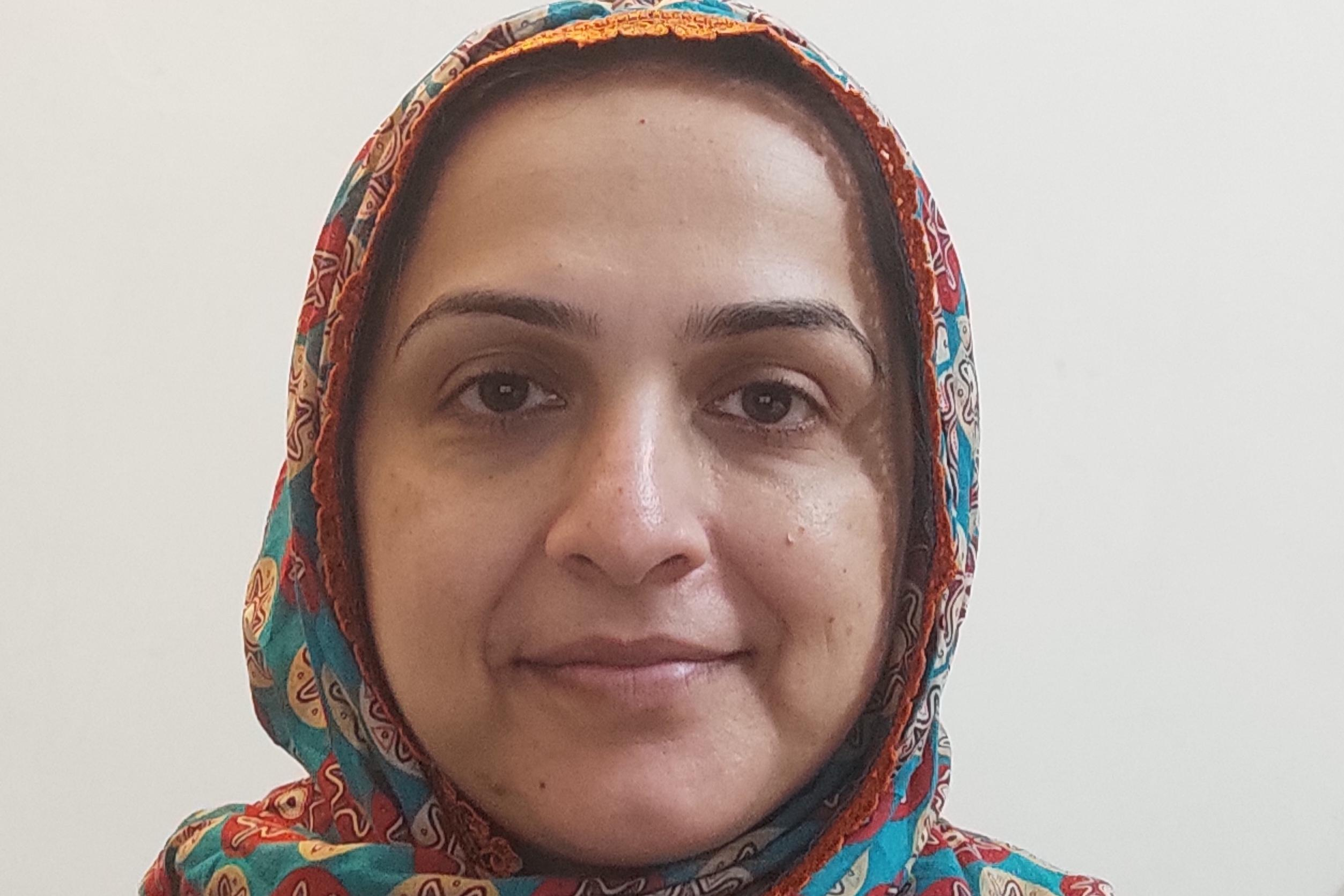Event date:
Sep 17 2021 2:30 pm

## The Very Basics of Lie Symmetry Methods For Solving Ordinary Differential Equations

Supervisor
Dr. Imran Naeem
Student
Mariam Siddiq Alvi
Venue
Zoom Meetings (Online)
Event
PhD Research Seminar
Abstract
The constant of integration in the solution of a differential equation is a member of the latter’s continuous symmetry group, a group of transformations that map one solution to another solution of the equation and also leave the equation invariant-- this crucial observation was made by the Norwegian mathematician Marius Sophus Lie in the second half of the nineteenth century. Lie aimed to construct a general theory of integration that would consolidate the existing disparate methods of integration. He successfully showed that knowledge of a symmetry group of a differential equation leads to quadrature or reduction in order. In particular, he introduced the use of the infinitesimal properties of a continuous symmetry group in his work. This seminar, comprising some rudimentary theory and a simple application in the form of an algorithm used to solve a first order ordinary differential equation, is meant to be an introduction to the very basics of Lie symmetry methods.

ZOOM Information:

Meeting ID: 984 9670 7254
Passcode: 490420